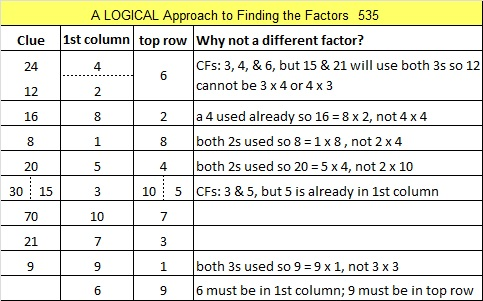# 535 and Level 6

535 is the hypotenuse of the Pythagorean triple 321-428-535. Can you find the greatest common factor of those three numbers?Print the puzzles or type the solution on this excel file: 10 Factors 2015-06-22

—————————————————————————————————

• 535 is a composite number.
• Prime factorization: 535 = 5 x 107
• The exponents in the prime factorization are 1 and 1. Adding one to each and multiplying we get (1 + 1)(1 + 1) = 2 x 2 = 4. Therefore 535 has exactly 4 factors.
• Factors of 535: 1, 5, 107, 535
• Factor pairs: 535 = 1 x 535 or 5 x 107
• 535 has no square factors that allow its square root to be simplified. √535 ≈ 23.130067—————————————————————————————————This site uses Akismet to reduce spam. Learn how your comment data is processed.